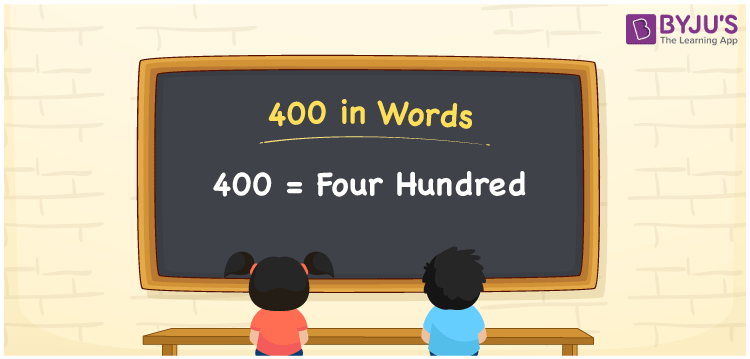# 400 in words

400 in words is written as Four Hundred. 400 represents the count or value. The article on Counting Numbers can give you an idea about count or counting. The number 400 is used in expressions that relate to money, days, distance, length, weight and so on. Let us consider an example for 400. “The Auto fare from my place to my office is Rupees Four Hundred”. Another example, “The primary school strength is 400 students.”

## 400 in English Words## How to Write 400 in Words?

We can convert 400 to words using a place value chart. The number 400 has 3 digits, so let’s make a chart that shows the place value up to 3 digits.

 Hundreds Tens Ones 4 0 0

Thus, we can write the expanded form as:

4 × Hundred + 0 × Ten + 0 × One

= 4 × 100 + 0 × 10 + 0 × 1

= 400

= Four Hundred.

400 is the natural number that is succeeded by 399 and preceded by 401.

400 in words – Four Hundred.

Is 400 an odd number? – No.

Is 400 an even number? – Yes.

Is 400 a perfect square number? – Yes.

Is 400 a perfect cube number? – No.

Is 400 a prime number? – No.

Is 400 a composite number? – Yes.

## Solved Example

1. Write the number 400 in expanded form

Solution: 4 × 100 + 0 × 10 + 0 × 1

We can write 400 = 400 + 0 + 0

= 4 × 100 + 0 × 10 + 0 × 1

## Frequently Asked Questions on 400 in words

Q1

### How to write 400 in words?

400 in words is written as Four Hundred.
Q2

### State True or False. 400 is divisible by 2?

True. 400 is divisible by 2. It is also divisible by 4, 5, 10 and 100.
Q3

### Is 400 a perfect square number?

Yes. 400 is a perfect square number. 20 times 20 is 400.